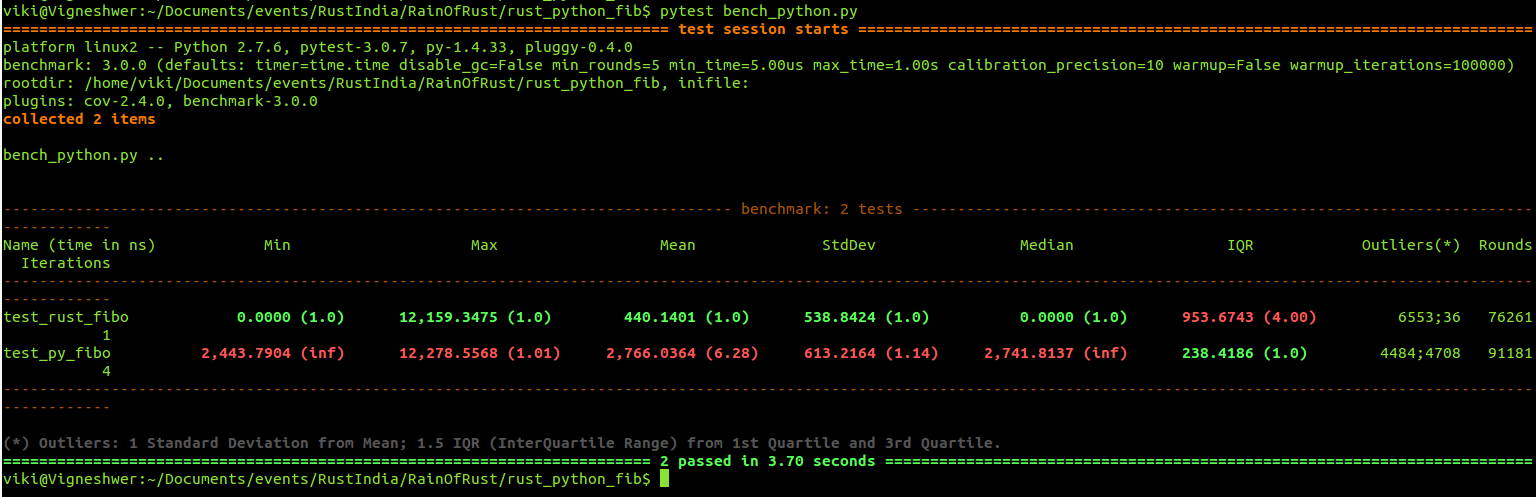# Creating python module in Rust

The objective of the blog post is to implement a python module using the state of art programming language Rust. The idea is to create an python module in Rust.

We will be using the rust-cpython project which makes it possible to execute Python code from Rust and vice-versa build a module in Rust for Python.

# Python module

In this section lets create a simple python function for example fibonacci sequence calculator. Follow the steps below to implement this code,

• Create a file named sample_fib.py and copy paste the content below.
``````#Define a function in python
def fib(n):

#If input is less than 2 return one
if n < 2:
return 1

#Assign the first two values of the series to be one
prev1 = 1
prev2 = 1

#Between the values 1 to the final input
for i in range(1, n):

next = prev1 + prev2
prev2 = prev1
prev1 = next

return prev1

#Execution starts here
if __name__ == '__main__':
print fib(3)
``````
• To run the code,
``````python sample_fib.py
``````

# Rust Implementation

Now lets implement the same python module in Rust and for this we would be using Rust-cpython library, which seamlessly allows the developer to build for Python 2 & 3 with few manipulation in the Cargo.toml file to download the crate.

• Create a new rust projects
``````cargo new rust_python_fib
``````

We are creating a library in Rust here.

• Enter the new project created:
``````cd rust_python_fib
``````
``````nano Cargo.toml
``````

The Cargo.toml should look something like this,

``````[package]
name = "python-rust-example"
version = "0.1.0"
authors = ["Vigneshwer <dvigneshwer@gmail.com>"]

[lib]
name = "example"
crate-type = ["dylib"]

[dependencies.cpython]
git = "https://github.com/dgrunwald/rust-cpython.git"
default-features = false
features = ["python27-sys"]

``````
• Go to ./src/lib.rs and edit the lib.rs file with the code below,
``````#[macro_use] extern crate cpython;

use cpython::{PyString, Python, PyResult};

//
fn fibo(py: Python, n : u64) -> PyResult<u64> {
if n < 2 {
return Ok(1)
}
let mut prev1 = 1;
let mut prev2 = 1;
for _ in 1..n {
let new = prev1 + prev2;
prev2 = prev1;
prev1 = new;
}
Ok(prev1)
}

// To build a Python compatible module we need an intialiser which expose the public interface
py_module_initializer!(example, initexample, PyInit_example, |py, m| {
// Expose our function fibo as `extern "C"`

// Initialiser s macro needs a Result<> as return value
Ok(())
});

``````
• Compile the project
``````cd .. && cargo build --release
``````
• Use the project in Python
``````cp ./target/release/libexample.so ./example.so

python
>>import example
>>print(example.fibo(4))
``````

# Benchmarking

Great if you have reached till here, we are almost done. Let’s take a step further to benchmark the performance of the newly create Rust-python module.

• Create a python file and open it in your favourite editor:
``````touch bench_python.py && subl bench_python.py
``````
• Copy and paster the code snippet below in the python script
``````#-- #########################
#-- Author: Vigneshwer.D
#-- Version: 1.0.0
#-- Date: 22 May 17
#-- #########################

# Importing modules
import example

# fibonacci implementation in Rust
def rust_fibo(val):
return example.fibo(val)

# fibonacci implementation in Python
def py_fibo(n):
if n < 2:
return 1

prev1 = 1
prev2 = 1

for i in range(1, n):
next = prev1 + prev2
prev2 = prev1
prev1 = next

return prev1

val =50

# benchmarking rust_fibo
def test_rust_fibo(benchmark):
benchmark(rust_fibo, val)

# benchmarking py_fibo
def test_py_fibo(benchmark):
benchmark(py_fibo, val)

if __name__ == '__main__':

# Series value
val =50

# Calling rust version
rust_val = rust_fibo(val)
print "Value predicted by rust_fibo: ", rust_val

# Calling python version
py_val = py_fibo(val)
print "Value predicted by py_fibo: ", py_val
``````
• To run the script:
``````python bench_python.py
``````
• To benchmark the performance:
``````pytest bench_python.py
``````

Note:

• To install the pytest framework:
``````sudo pip install pytest-benchmark
``````

This should give you an output simillar to:# Conclusion

Rust is great language to build python module and has sea of oppurtunities in terms of getting high performance. I am personally excited about building numpy functions in Rust and benchmark them.

Ref:

Written on April 15, 2017